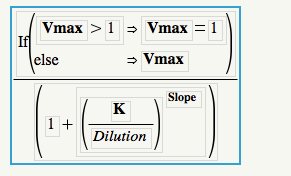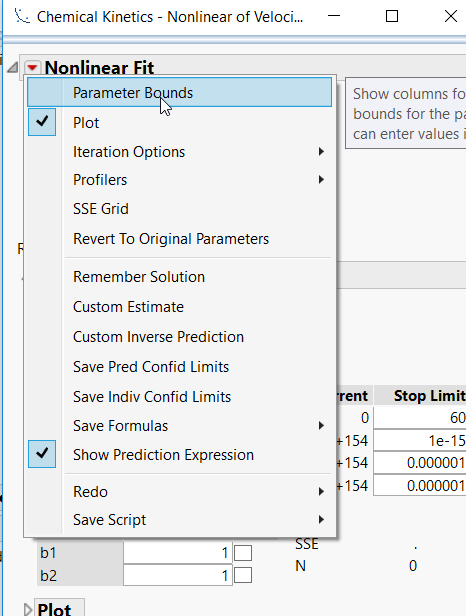Choose Language Hide Translation Bar
Highlighted

## Can I Define Nonlinear Parameter Range?

I have the following predictor formula in a column

``VMax / (1 + (K / :Dilution) ^ Slope)``

where Vmax, K and Slope are all parameters.

This formula describes a biological assay where Vmax cannot be greater than 100% (both mathematically and biologically). I would like to constrain the range for Vmax between 0 and 100 to ensure K and Slope are accurate. Any help would be fantastic.

Thanks

2 REPLIES 2
HighlightedJeff_PerkinsonCommunity Manager

## Re: Can I Define Nonlinear Parameter Range?

There may be a different way to do this, but I think you can put the constraint into your predictor formula.

Like this:

``If( Vmax > 1, Vmax = 1, Vmax ) / (1 + (K / :Dilution) ^ Slope)``-Jeff
Highlighted

## Re: Can I Define Nonlinear Parameter Range?

In the Nonlinear platform you can specify parameter bounds and this will constrain the parameters to stay within those bounds during the model fitting.Article Labels

There are no labels assigned to this post.